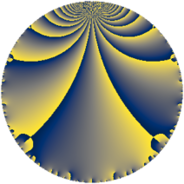Properties

 Label 1859.4.jLevel $1859$ Weight $4$ Character orbit 1859.j Rep. character $\chi_{1859}(23,\cdot)$ Character field $\Q(\zeta_{6})$ Dimension $768$ Sturm bound $728$

Related objects

Defining parameters

 Level: $$N$$ $$=$$ $$1859 = 11 \cdot 13^{2}$$ Weight: $$k$$ $$=$$ $$4$$ Character orbit: $$[\chi]$$ $$=$$ 1859.j (of order $$6$$ and degree $$2$$) Character conductor: $$\operatorname{cond}(\chi)$$ $$=$$ $$13$$ Character field: $$\Q(\zeta_{6})$$ Sturm bound: $$728$$

Dimensions

The following table gives the dimensions of various subspaces of $$M_{4}(1859, [\chi])$$.

Total New Old
Modular forms 1120 768 352
Cusp forms 1064 768 296
Eisenstein series 56 0 56

Trace form

 $$768 q + 12 q^{3} + 1528 q^{4} - 90 q^{6} + 36 q^{7} - 3420 q^{9} + O(q^{10})$$ $$768 q + 12 q^{3} + 1528 q^{4} - 90 q^{6} + 36 q^{7} - 3420 q^{9} + 56 q^{10} + 132 q^{12} - 328 q^{14} - 6076 q^{16} - 138 q^{17} - 492 q^{19} - 540 q^{20} + 44 q^{22} + 42 q^{23} - 720 q^{24} - 19492 q^{25} + 504 q^{27} + 714 q^{28} + 246 q^{29} + 64 q^{30} - 144 q^{32} + 136 q^{35} + 13510 q^{36} - 630 q^{37} - 132 q^{38} - 216 q^{40} - 126 q^{41} + 176 q^{42} - 588 q^{43} + 570 q^{45} + 1590 q^{46} - 1934 q^{48} + 19388 q^{49} - 1290 q^{50} + 2600 q^{51} - 836 q^{53} + 528 q^{54} + 440 q^{55} - 148 q^{56} + 1128 q^{58} - 504 q^{59} - 590 q^{61} - 2028 q^{62} - 1884 q^{63} - 50052 q^{64} - 3256 q^{66} - 3396 q^{67} - 2046 q^{68} - 12 q^{69} + 1014 q^{71} + 1062 q^{72} + 1268 q^{74} - 6140 q^{75} - 7872 q^{76} + 1232 q^{77} + 2960 q^{79} + 558 q^{80} - 30240 q^{81} + 4612 q^{82} - 696 q^{84} - 4662 q^{85} - 2240 q^{87} - 924 q^{88} - 1014 q^{89} - 2860 q^{90} + 796 q^{92} + 4092 q^{93} - 4406 q^{94} + 744 q^{95} + 5070 q^{97} + 8550 q^{98} + O(q^{100})$$

Decomposition of $$S_{4}^{\mathrm{new}}(1859, [\chi])$$ into newform subspaces

The newforms in this space have not yet been added to the LMFDB.

Decomposition of $$S_{4}^{\mathrm{old}}(1859, [\chi])$$ into lower level spaces

$$S_{4}^{\mathrm{old}}(1859, [\chi]) \cong$$ $$S_{4}^{\mathrm{new}}(13, [\chi])$$$$^{\oplus 4}$$$$\oplus$$$$S_{4}^{\mathrm{new}}(143, [\chi])$$$$^{\oplus 2}$$$$\oplus$$$$S_{4}^{\mathrm{new}}(169, [\chi])$$$$^{\oplus 2}$$